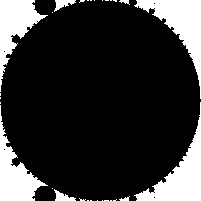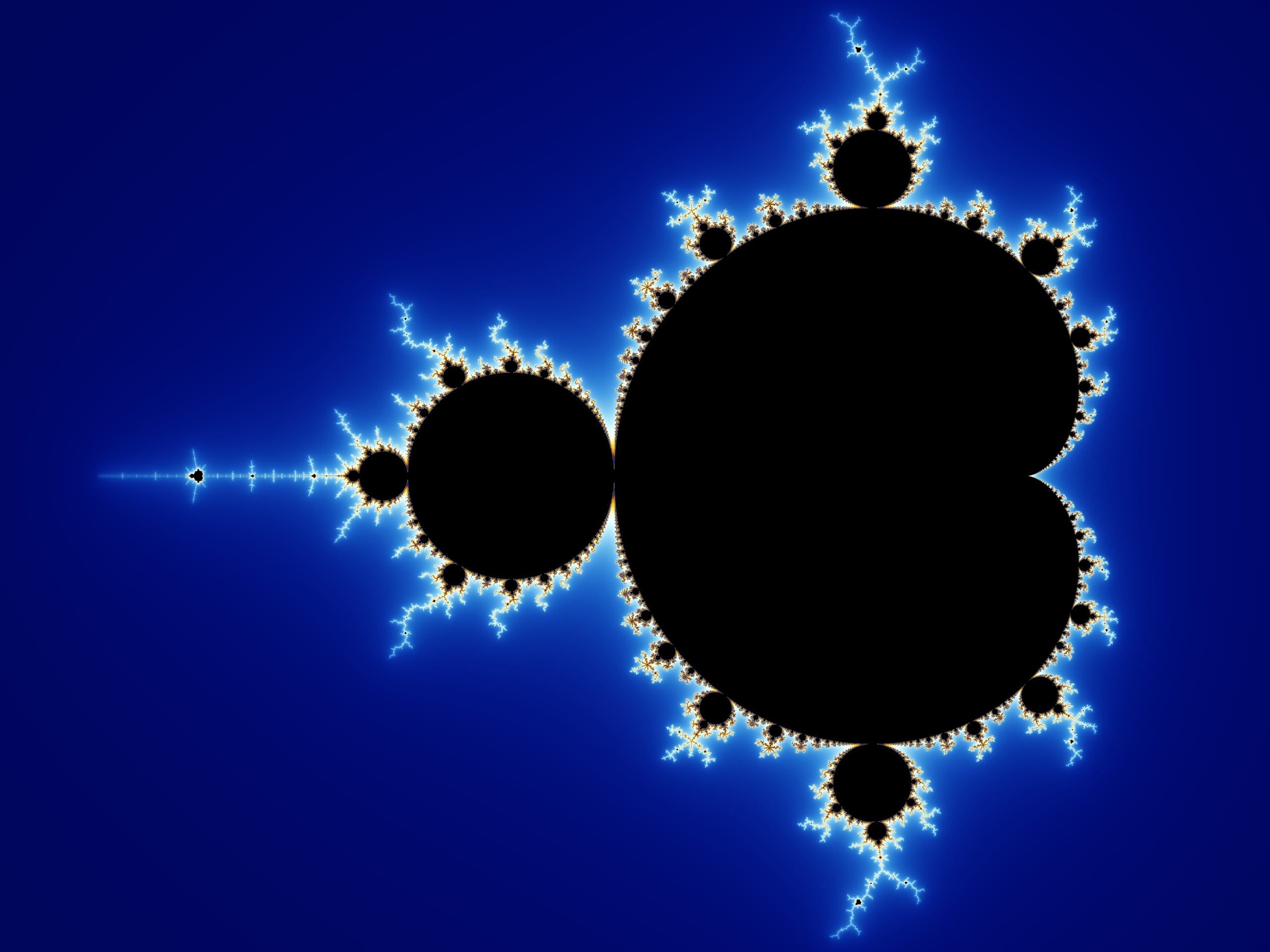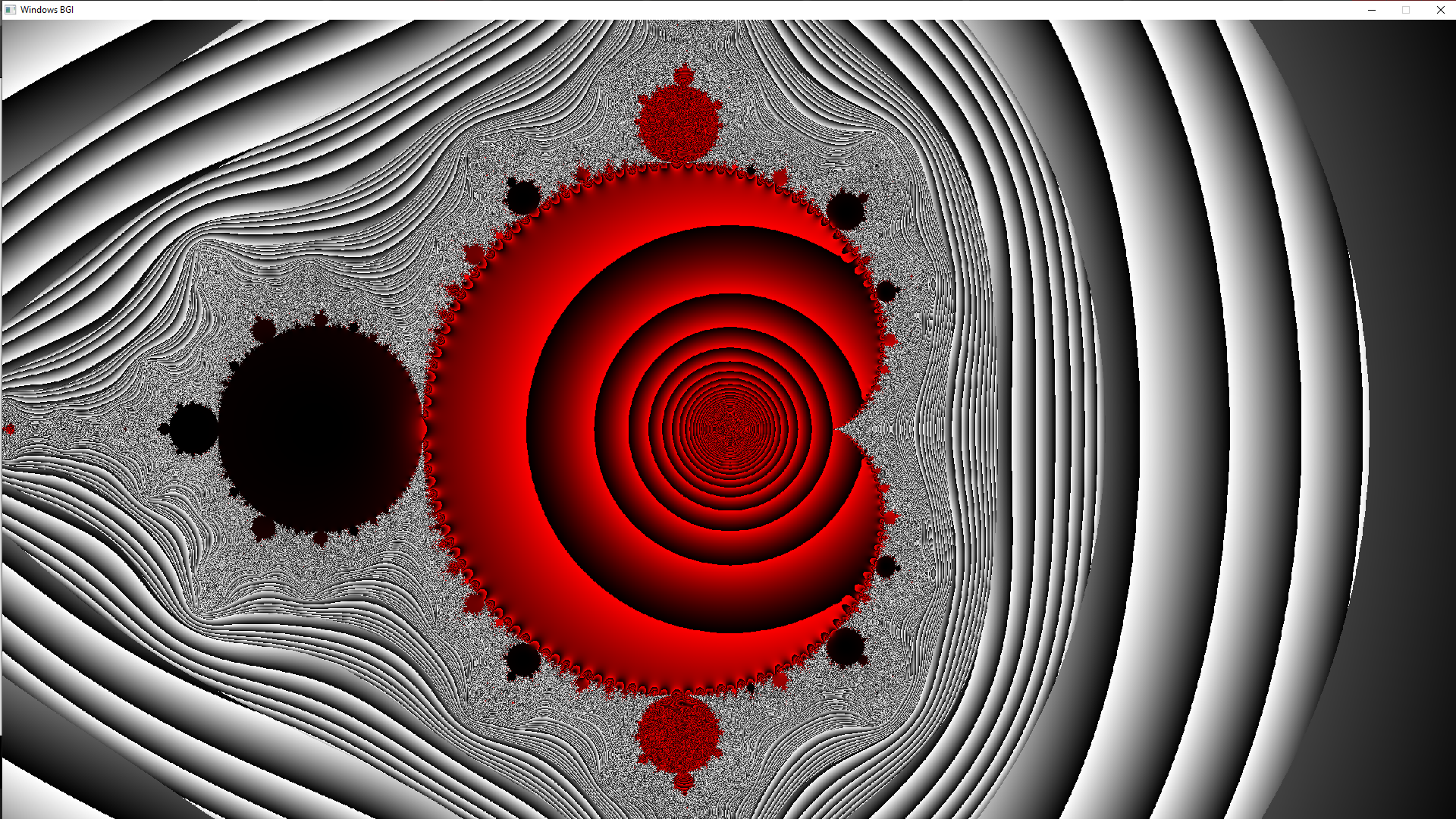# Mandlebrot Set in C/C++ Using Graphics

### Fractals

A Fractal is a never-ending pattern. Fractals are infinitely complex patterns that are self-similar across different scales. They are created by repeating a simple process over and over in an ongoing feedback loop. Mathematically fractals can be explained as follows.

• The location of a point on a screen is fed into an equation as its initial solution and the equation is iterated a large number of times.
• If that equation tends to zero (i.e. the value at the end of the iterations is smaller than the initial value), the point is coloured black.
• If the equation tends to infinity (i.e. the final value is larger than the initial value) then depending on the rate of increase (i.e. the rate at which the value tends to infinity), the pixel is painted with an appropriate colour.
• ### Defining Mandlebrot

The Mandelbrot set is the set of complex numbers c for which the functiondoes not diverge when iterated from z=0, i.e., for which the sequence, etc., remains bounded in absolute value. In simple words, Mandelbrot set is a particular set of complex numbers which has a highly convoluted fractal boundary when plotted.Implementation

 `#include ` `#include ` `#include ` ` `  `// Defining the size of the screen. ` `#define Y 1080 ` `#define X 1920 ` ` `  `// Recursive function to provide the iterative every 100th ` `// f^n (0) for every pixel on the screen. ` `int` `Mandle(``double` `_Complex c, ` `           ``double` `_Complex t = 0, ` `           ``int` `counter = 0) ` `{ ` ` `  `    ``// To eliminate out of bound values. ` `    ``if` `(cabs(t) > 4) { ` `        ``putpixel(creal(c) * Y / 2 + X / 2, ` `                 ``cimag(c) * Y / 2 + Y / 2, ` `                 ``COLOR(128 - 128 * cabs(t) / cabs(c), ` `                       ``128 - 128 * cabs(t) / cabs(c), ` `                       ``128 - 128 * cabs(t) / cabs(c))); ` `        ``return` `0; ` `    ``} ` ` `  `    ``// To put about the end of the fractal, ` `    ``// the higher the value of the counter, ` `    ``// The more accurate the fractal is generated, ` `    ``// however, higher values cause ` `    ``// more processing time. ` `    ``if` `(counter == 100) { ` `        ``putpixel(creal((c)) * Y / 2 + X / 2, ` `                 ``cimag((c)) * Y / 2 + Y / 2, ` `                 ``COLOR(255 * (cabs((t * t)) ` `                              ``/ cabs((t - c) * c)), ` `                       ``0, 0)); ` `        ``return` `0; ` `    ``} ` ` `  `    ``// recursively calling Mandle with increased counter ` `    ``// and passing the value of the squares of t into it. ` `    ``Mandle(c, cpow(t, 2) + c, counter + 1); ` ` `  `    ``return` `0; ` `} ` ` `  `int` `MandleSet() ` `{ ` ` `  `    ``// Calling Mandle function for every ` `    ``// point on the screen. ` `    ``for` `(``double` `x = -2; x < 2; x += 0.0015) { ` `        ``for` `(``double` `y = -1; y < 1; y += 0.0015) { ` `            ``double` `_Complex temp = x + y * _Complex_I; ` `            ``Mandle(temp); ` `        ``} ` `    ``} ` `    ``return` `0; ` `} ` ` `  `int` `main() ` `{ ` `    ``initwindow(X, Y); ` `    ``MandleSet(); ` `    ``getch(); ` `    ``closegraph(); ` `    ``return` `0; ` `} `

OutputMy Personal Notes arrow_drop_upCheck out this Author's contributed articles.

If you like GeeksforGeeks and would like to contribute, you can also write an article using contribute.geeksforgeeks.org or mail your article to contribute@geeksforgeeks.org. See your article appearing on the GeeksforGeeks main page and help other Geeks.

Please Improve this article if you find anything incorrect by clicking on the "Improve Article" button below.

Article Tags :

1

Please write to us at contribute@geeksforgeeks.org to report any issue with the above content.